## How to sum unique values based on criteria in Excel?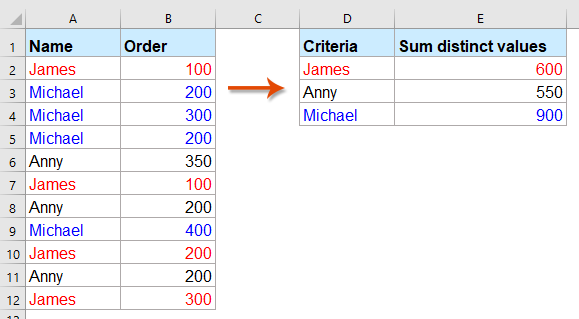For example, I have a range of data which contains Name and Order columns, now, to sum only unique values in Order column based on the Name column as following screenshot shown. How to solve this task quickly and easily In Excel?

Sum unique values based on one or more criteria with array formulas

#### Sum unique values based on one or more criteria with array formulas

To sum only unique values based on criteria in another column, please use the below formula:

1. Please copy or enter the following array formula into a blank cell:

=SUM(IF(FREQUENCY(IF(\$A\$2:\$A\$12=D2,MATCH(\$B\$2:\$B\$12,\$B\$2:\$B\$12,0)),ROW(\$B\$2:\$B\$12)-ROW(\$B\$2)+1),\$B\$2:\$B\$12))

Note: In the above formula, A2:A12 is the column data contains the condition value, D2 is the condition that you want to sum values based on, B2:B12 is the column contains the values that you want to sum.

2. Then, press Ctrl + Shift + Enter keys together to get the first total result, and then, select this formula cell and drag the fill handle down to the cells to get all total values for each item. See screenshot: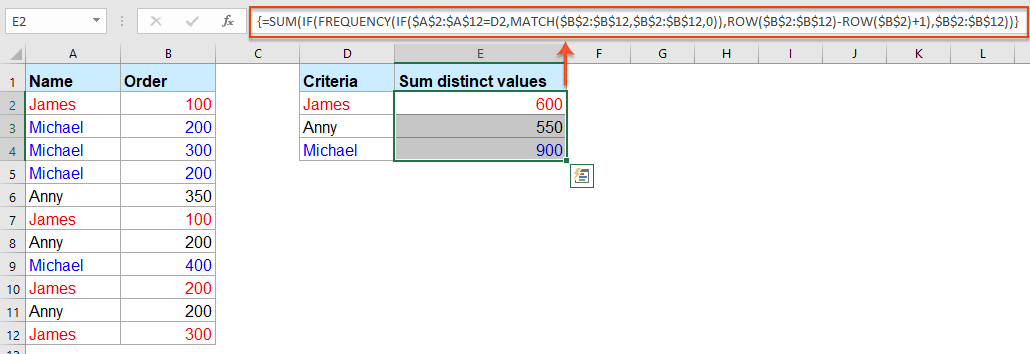Tips: If you need to sum all the unique values based on two criteria, please apply the below formula:

=SUM(IF(FREQUENCY(IF(\$A\$2:\$A\$12=E2,IF(\$B\$2:\$B\$12=F2,MATCH(\$C\$2:\$C\$12,\$C\$2:\$C\$12,0))),ROW(\$C\$2:\$C\$12)-ROW(\$C\$2)+1),\$C\$2:\$C\$12))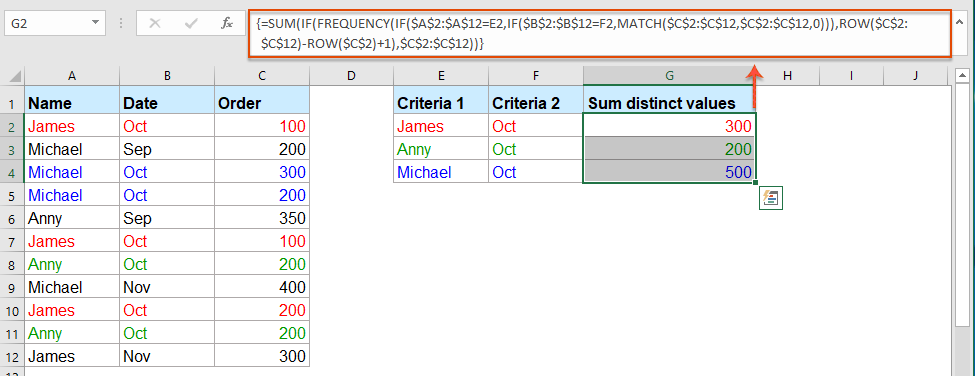#### More relative articles:

• Sum Multiple Columns Based On Single Criteria In Excel
• In Excel, you may always need to sum multiple columns based on one criteria. For example, I have a range of data as following screenshot shown, now, I want to get the total values of KTE in three months - Jan, Feb and Mar.
• Vlookup And Sum Matches In Rows Or Columns In Excel
• Using vlookup and sum function helps you quickly find out the specified criteria and sum the corresponding values at the same time. In this article, we are going to show you two methods to vlookup and sum the first or all matched values in rows or columns in Excel.
• Sum Values Based On Month And Year In Excel
• If you have a range of data, column A contains some dates and column B has the number of orders, now, you need to sum the numbers based on month and year from another column. In this case, I want to calculate the total orders of January 2016 to get the following result. And this article, I will talk about some tricks to solve this job in Excel.
• Sum Values Based On Text Criteria In Excel
• In Excel, have you ever tried to sum values based on another column of text criteria? For example, I have a range of data in worksheet as following screenshot shown, now, I want to add up all the numbers in column B corresponding with the text values in column A that meet a certain criterion, such as sum the numbers if the cells in column A contains KTE.
• Sum Values Based On Selection Of Drop-Down List In Excel
• As below screenshot shown, you have a table contains a Category column and an Amount column, and you have created a data validation drop-down list which contains all the categories. When selecting any kind of category from the drop-down list, you want to sum up all corresponding cell values in Column B and populate the result in a specified cell. For example, when selecting category CC from the drop-down list, you need to sum values in cell B5 and B8 and get the total number 40+70=110. How to achieve it? Method in this article can help you.

## The Best Office Productivity Tools

### Kutools for Excel Solves Most of Your Problems, and Increases Your Productivity by 80%

• Super Formula Bar (easily edit multiple lines of text and formula); Reading Layout (easily read and edit large numbers of cells); Paste to Filtered Range...
• Merge Cells/Rows/Columns and Keeping Data; Split Cells Content; Combine Duplicate Rows and Sum/Average... Prevent Duplicate Cells; Compare Ranges...
• Select Duplicate or Unique Rows; Select Blank Rows (all cells are empty); Super Find and Fuzzy Find in Many Workbooks; Random Select...
• Exact Copy Multiple Cells without changing formula reference; Auto Create References to Multiple Sheets; Insert Bullets, Check Boxes and more...
• Favorite and Quickly Insert Formulas, Ranges, Charts and Pictures; Encrypt Cells with password; Create Mailing List and send emails...
• Extract Text, Add Text, Remove by Position, Remove Space; Create and Print Paging Subtotals; Convert Between Cells Content and Comments...
• Super Filter (save and apply filter schemes to other sheets); Advanced Sort by month/week/day, frequency and more; Special Filter by bold, italic...
• Combine Workbooks and WorkSheets; Merge Tables based on key columns; Split Data into Multiple Sheets; Batch Convert xls, xlsx and PDF...
• Pivot Table Grouping by week number, day of week and more... Show Unlocked, Locked Cells by different colors; Highlight Cells That Have Formula/Name...### Office Tab - brings tabbed interface to Office, and make your work much easier

• Enable tabbed editing and reading in Word, Excel, PowerPoint, Publisher, Access, Visio and Project.
• Open and create multiple documents in new tabs of the same window, rather than in new windows.
• Increases your productivity by 50%, and reduces hundreds of mouse clicks for you every day!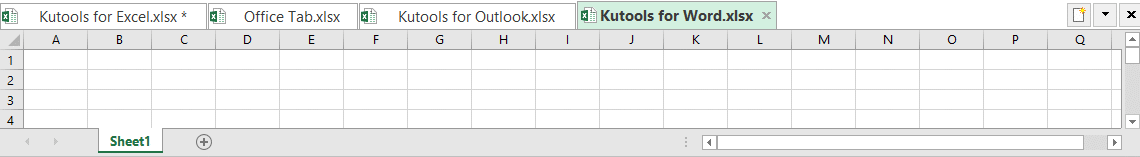No ratings yet. Be the first to rate!
This comment was minimized by the moderator on the site
I have column "A" listed with various Part nos. Say 50 parts. Each of these 50 parts will be repeated more than once. I column B where in for each of these part nos stock qty say for particular part no 1000 is the qty. This 1000 Qty is shown againt that particular part no in whichever cell it comes in column 'A".

The problem i am facing is sum total of column B is not correct as stock qty is shown n-number of times that particular part finds place in column A.

How do i avoid totaling up this repeated stock qty of one unique part. Say part no P1001 stock is 1000nos. If delivery date of this part P1001 is

Part no..Date.............Qty......Stock
P1001....05-10-22 .....150......1000
P1001....06-10-22......200.....1000
P1001....07-10-22.......250.....1000

in the above table stock is only 1000 but it shows against each delivery date the same stock qty. But when i want to sum the total stock of all part nos it should not take 1000 3 times but only 1 time. How do this?
This comment was minimized by the moderator on the site
Hello, Pugazh
Did you want to sum only the unique stock based on the part number?
Or you can give your problem as a creenshot here, so that, we can understand more clearly.
Thank you!
This comment was minimized by the moderator on the site
Hi,

I was wondering if there was a formula for three criteria? Or if there's a process/logic to follow for more than two criteria ?

Many thanks,
Gus
This comment was minimized by the moderator on the site
Hello, Gus,
If there are three criteria of your data, to sum the unique values, please apply the below array formula:
``=SUM(IF(FREQUENCY(IF(\$A\$2:\$A\$10=F2,IF(\$B\$2:\$B\$10=G2,IF(\$C\$2:\$C\$10=H2,MATCH(\$D\$2:\$D\$10,\$D\$2:\$D\$10,0)))),ROW(\$D\$2:\$D\$10)-ROW(\$C\$2)+1),\$D\$2:\$D\$10))``

After inserting the formula, please press press Ctrl + Shift + Enter keys together to get the result. see below screenshot: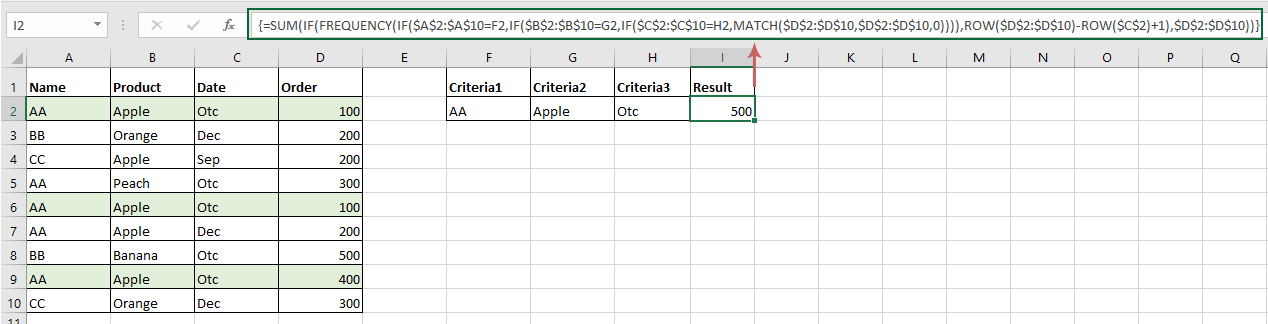Please have a try, thank you!
This comment was minimized by the moderator on the site
Hi,
I would like to sum based on two unique criteria, is this possible?
In the second example, rather than James and October being the specific criteria, James would stay the specific element, and the formula would sum based on the name and the month being unique.
Many thanks
Louise
This comment was minimized by the moderator on the site
Hola me podrias ayudar deseo sumar o contar solo valores unicos de fechas especificas me puedes ayudar ya que la formula =SUM(IF(FREQUENCY(IF(\$A\$2:\$A\$12=D2,MATCH(\$B\$2:\$B\$12,\$B\$2:\$B\$12,0)),ROW(\$B\$2:\$B\$12)-ROW(\$B\$2)+1),\$B\$2:\$B\$12)) me sirve pero como esta en ingles no se como colocarla, te agradezco mucho
This comment was minimized by the moderator on the site
Hello Jorge,

Formula: =SUM(IF(FREQUENCY(IF(\$A\$2:\$A\$12=E2,IF(\$B\$2:\$B\$12=F2,MATCH(\$C\$2:\$C\$12,\$C\$2:\$C\$12,0))),ROW(\$C\$2:\$C\$12)-ROW(\$C\$2)+1),\$C\$2:\$C\$12))

Translate the English version formula into the Spanish version:
=SUMA(SI(FRECUENCIA(SI(\$A\$2:\$A\$12=E2,SI(\$B\$2:\$B\$12=F2,COINCIDIR(\$C\$2:\$C\$12,\$C\$2:\$C\$12,0))),FILA(\$C\$2:\$C\$12)-FILA(\$C\$2)+1),\$C\$2:\$C\$12))

Sincerely,
Mandy
This comment was minimized by the moderator on the site
This doesn't actually work as I would have expected. The formula is only counting the unique criteria.

James had 700 units ordered in the first example, yet the total says 600.

The formula won't count his orders that contain the same qty because they are unique. Is there a way to modify this formula so you can get an actual total of all of James' orders?

The second formula has the same issue. James ordered 400 units, not 300 as shown in the summary.
This comment was minimized by the moderator on the site
Hello, kc, May be the below article can solve your problem, please try:https://www.extendoffice.com/documents/excel/2471-excel-sumif-multiple-criteria.html
There are no comments posted here yet